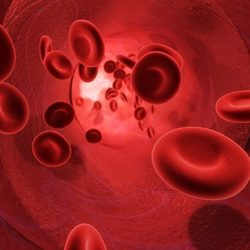Home  ›  Fitness and Exercise  ›  Calculators  ›  Heart Rate and Percent VO2max Conversion Calculator

# Heart Rate and Percent VO2max Conversion CalculatorThe calculators on this page allow you to determine your exercise intensity level as a percentage of your maximal oxygen consumption (VO2max) from your known heart rate, or vice versa. To determine your actual VO2max itself, you would ideally need to perform a VO2max maximal physical exertion test in a controlled setting with sophisticated laboratory equipment (read more about VO2 and VO2max). However, without actually knowing what your VO2max is you can still convert your exercise intensity level from a heart rate to a percentage of VO2max, and vice versa, since there is a known linear relationship between the two.

Heart Rate to Percent VO2max Conversion Calculator

Age (in years)

Heart Rate
(in beats/minute)

Percent VO2max to Heart Rate Conversion Calculator

Age (in years)

% of VO2max

## Equation

The experimental data on which these calculators are based covers a heart rate range from between 63% to 92% of maximum, which in turn corresponds to between 40% and 85% of VO2max. The calculators will allow you to convert any heart rate between 63% and 102% of your maximum heart rate to a percentage of your VO2max, or any percentage of VO2max from between 40% to 100% to a heart rate. Therefore, any determination of VO2max from a heart rate above 92%, or any determination of heart rate from a percentage of VO2max above 85%, is based on an extrapolation (i.e. an assumption that the data correlation holds outside the limits of the gathered data points) of the experimental data rather than an interpolation. Since the data correlation is not expected to remain valid below the lower limits of the gathered data points the calculators will not provide conversions for a heart rate lower than 63% of maximum or a percentage of VO2max lower than 40%.

The equation on which these calculators are based is shown below. It is a linear regression formula derived by ShapeSense.com from experimental data gathered by DP Swain, KS Abernathy, CS Smith, SJ Lee, and SA Bunn for their study titled "Target heart rates for the development of cardiorespiratory fitness."

• %MHR = 0.6463 x %VO2max + 37.182

where

%MHR = Percentage of Maximum Heart Rate
%VO2max = Percentage of Maximal Oxygen Consumption

Swain DP, Abernathy KS, Smith CS, Lee SJ, Bunn SA. Target heart rates for the development of cardiorespiratory fitness. Med Sci Sports Exerc. January 1994. 26(1): 112–116.